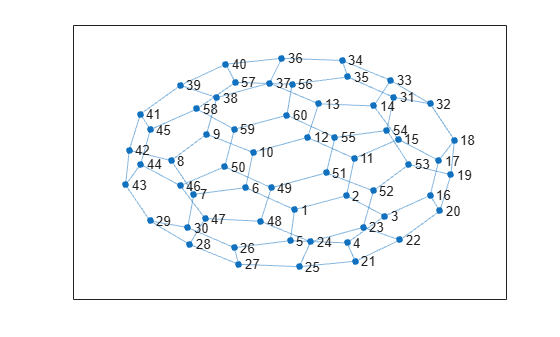# neighbors

## 语法

``N = neighbors(G,nodeID)``

## 说明

``N = neighbors(G,nodeID)` 返回通过边连接到由 `nodeID` 指定的节点的所有节点的节点 ID。`

## 示例

```G = graph(bucky); plot(G)````N = neighbors(G,10)`
```N = 3×1 6 9 12 ```